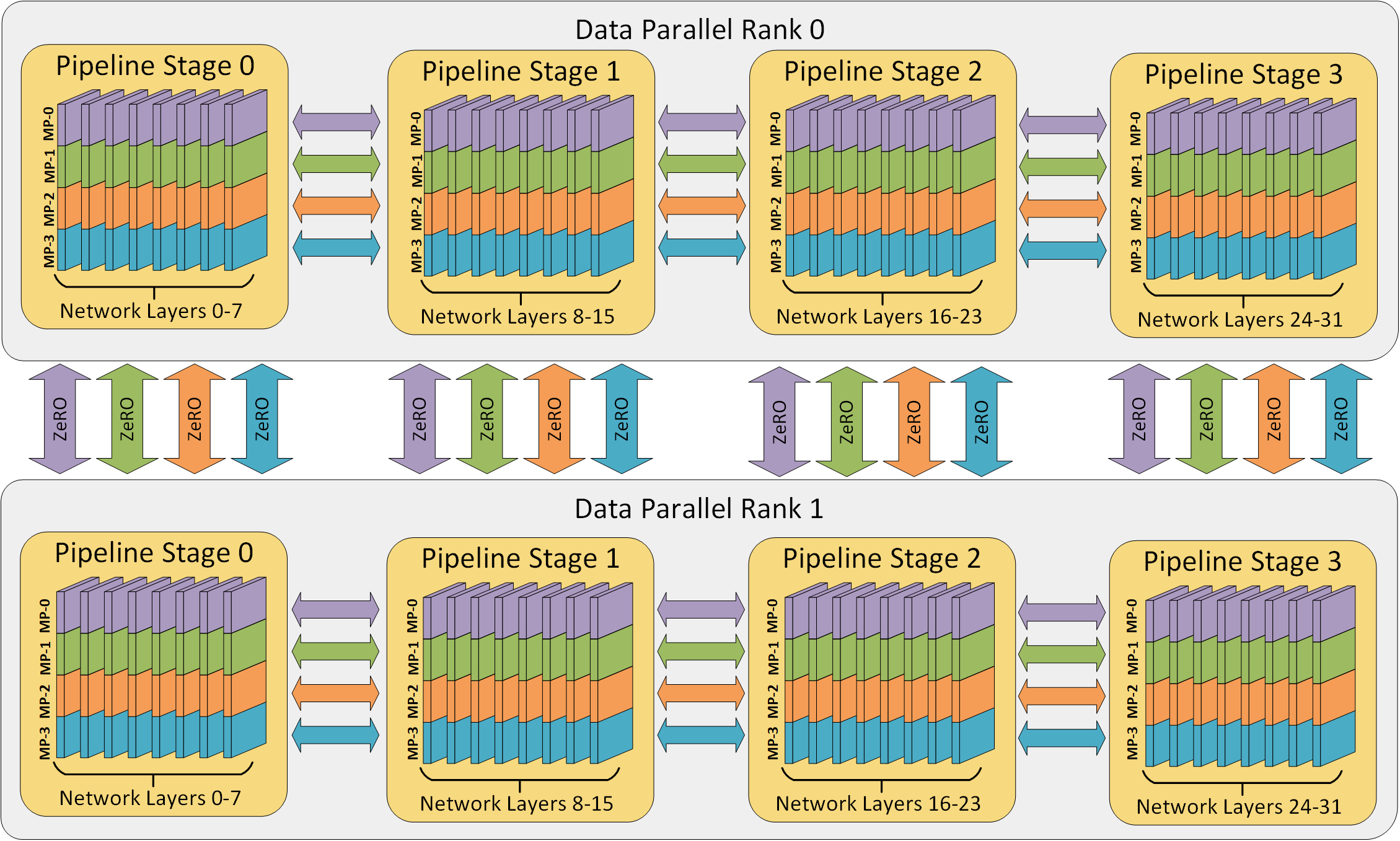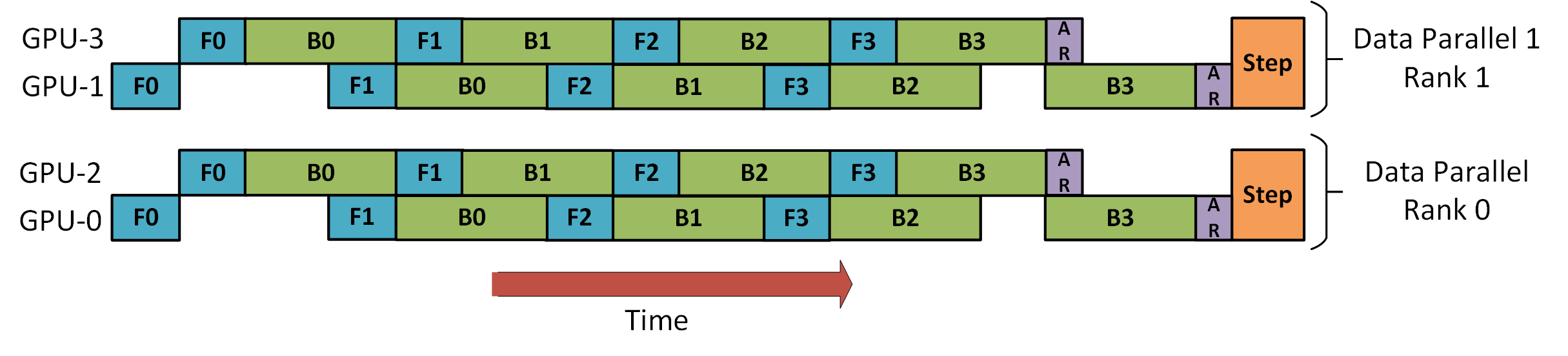# Pipeline Parallelism

DeepSpeed v0.3 includes new support for pipeline parallelism! Pipeline parallelism improves both the memory and compute efficiency of deep learning training by partitioning the layers of a model into stages that can be processed in parallel. DeepSpeed’s training engine provides hybrid data and pipeline parallelism and can be further combined with model parallelism such as Megatron-LM. An illustration of 3D parallelism is shown below. Our latest results demonstrate that this 3D parallelism enables training models with over a trillion parameters.DeepSpeed uses gradient accumulation to extract pipeline parallelism (shown below). Each batch of training data is divided into micro-batches that can be processed in parallel by the pipeline stages. Once a stage completes the forward pass for a micro-batch, the activation memory is communicated to the next stage in the pipeline. Similarly, as the next stage completes its backward pass on a micro-batch, the gradient with respect to the activation is communicated backwards through the pipeline. Each backward pass accumulates gradients locally. Next, all data parallel groups perform reductions of the gradients in parallel. Lastly, the optimizer updates the model weights.

Below is an illustration of how DeepSpeed will train a batch with eight micro-batches using hybrid two-way data parallelism and two-stage pipeline parallelism. GPUs 0 and 2 are arranged in a pipeline and will alternate forward (F) and backward (B) passes. They will then all-reduce (AR) gradients with their data parallel counterparts, GPUs 1 and 3, respectively. Finally, the two pipeline stages update their model weights.## Getting Starting with Pipeline Parallelism

DeepSpeed strives to accelerate and simplify the process of pipeline parallel training. This section provides first steps with hybrid data and pipeline parallel training by preparing `torchvision`’s AlexNet model.

### Expressing Pipeline Models

Pipeline parallelism requires models to be expressed as a sequence of layers. In the forward pass, each layer consumes the output of the previous layer. In fact, there is no need to specify a `forward()` for a pipeline parallel model! The forward pass of a pipeline parallel model implicitly takes the form:

``````def forward(self, inputs):
x = inputs
for layer in self.layers:
x = layer(x)
return x
``````

PyTorch’s `torch.nn.Sequential` is a convenient container for expressing pipeline parallel models and can be parallelized by DeepSpeed with no modification:

``````net = nn.Sequential(
nn.Linear(in_features, hidden_dim),
nn.ReLU(inplace=True),
nn.Linear(hidden_dim, out_features)
)
from deepspeed.pipe import PipelineModule
net = PipelineModule(layers=net, num_stages=2)
``````

`PipelineModule` uses its `layers` argument as the sequence of layers that comprise the model. After initialization, `net` is divided into two pipeline stages and its layers moved to the corresponding GPUs. If more than two GPUs are present, DeepSpeed will also use hybrid data parallelism.

Note: The total number of GPUs must be divisible by the number of pipeline stages.

Note: For large model training, see memory-efficient model construction.

### AlexNet

Let’s look at an abbreviated implementation of `torchvision`’s AlexNet:

``````class AlexNet(nn.Module):
def __init__(self, num_classes=1000):
super(AlexNet, self).__init__()
self.features = nn.Sequential(
...
nn.MaxPool2d(kernel_size=3, stride=2),
)
self.classifier = nn.Sequential(
nn.Dropout(),
...
nn.Linear(4096, num_classes),
)

def forward(self, x):
x = self.features(x)
x = self.avgpool(x)
x = torch.flatten(x, 1)
x = self.classifier(x)
return x
``````

`AlexNet` is mostly a composition of several `Sequential` submodules. We can turn this into a `PipelineModule` by flattening its submodules into a single sequence of layers:

``````class AlexNetPipe(AlexNet):
def to_layers(self):
layers = [
*self.features,
self.avgpool,
lambda x: torch.flatten(x, 1),
*self.classifier
]
return layers

from deepspeed.pipe import PipelineModule
net = AlexNetPipe()
net = PipelineModule(layers=net.to_layers(), num_stages=2)
``````

Note: the `lambda` in the middle of `layers` above is not a `torch.nn.Module` type. Any object that implements `__call__()` can be a layer in a `PipelineModule`: this allows for convenient data transformations in the pipeline.

### Inputs and Outputs

Following `torch.nn.Sequential`, the inputs and outputs of each layer must be either a single `torch.Tensor` or a `tuple` of tensors. In practice, some models may need to modify their forward pass to pack and unpack arguments to `forward()`. Consider an abbreviated implementation of a stack of Transformer blocks:

``````class TransformerBlock(nn.Module)
...
return output
...

stack = [ TransformerBlock() for _ in range(num_layers) ]
``````

Two modifications to `TransformerBlock` are required:

1. The arguments must be collected into a `tuple`.
2. `mask` must also be returned from `forward()` to pass to the next layer.

These modifications can be accomplished with a short subclass:

``````class TransformerBlockPipe(TransformerBlock)
def forward(self, inputs):
stack = [ TransformerBlockPipe() for _ in range(num_layers) ]
``````

### Training Loops

Pipeline parallelism interleaves forward and backward passes, and thus the training loop cannot be divided into separate stages of `forward()`, `backward()` and `step()`. Instead, DeepSpeed’s pipeline engine provides a `train_batch()` method that advances the pipeline engine until the next batch of training data is consumed and the model weights updated.

``````train_iter = iter(train_loader)
loss = engine.train_batch(data_iter=train_iter)
``````

The above `train_batch()` example is equivalent to the following with traditional data parallel DeepSpeed:

``````train_iter = iter(train_loader)
batch = next(data_iter)
loss = engine(batch)
engine.backward(loss)
engine.step()
``````

### Dealing with Data

Data parallel training typically has each worker perform IO independently at the start of each batch. However, in a pipeline parallel environment, only the first stage uses the input data, and only the last stage uses labels for loss calculation.

Note: The pipeline engine expects data loaders to return a `tuple` of two items. The first returned item is the input batch data, and the second item is the data to be used in the loss calculation. As before, inputs and labels should be either `torch.Tensor` type or a `tuple` of tensors.

For convenience, the DeepSpeed pipeline engine can construct a distributed data loader when a dataset is provided to `deepspeed.initialize()`. DeepSpeed handles the rest of the complexity of data loading, and so the pipeline training loop becomes:

``````engine, _, _, _ = deepspeed.initialize(
args=args,
model=net,
model_parameters=[p for p in net.parameters() if p.requires_grad],
training_data=cifar_trainset())

for step in range(args.steps):
loss = engine.train_batch()
``````

Of course, DeepSpeed will work with any data loader that you wish to use. Data loaders should be constructed by the first and last stages in the pipeline. Each worker should load micro-batches of size `engine.train_micro_batch_size_per_gpu()` and will be queried a total of `engine.gradient_accumulation_steps()` times per `train_batch()`.

Watch out! The pipeline engine pulls data from an iterator instead of iterating over it. It’s critical that the data stream does not empty in the middle of a training batch. Each invocation of `train_batch()` will pull a total of `engine.gradient_accumulation_steps()` micro-batches of data from the data iterator.

DeepSpeed provides a convenience class `deepspeed.utils.RepeatingLoader` that simply wraps an iterable such as a data loader and restarts it whenever the end is reached:

``````train_loader = deepspeed.utils.RepeatingLoader(train_loader)
for step in range(args.steps):
loss = engine.train_batch(data_iter=train_iter)
``````

The performance of pipeline parallel training strongly relies on load balance. DeepSpeed provides several mechanisms for partitioning the model across GPUs. These strategies can be set with the `partition_method` keyword argument to `PipelineModule`. Here are partitioning methods currently provided by DeepSpeed:

• `partition_method="parameters"` (default) balances the number of trainable parameters on each pipeline stage . This is especially useful in memory-constrained environments and when the size of a layer is proportional to the computation time.
• `partition_method="type:[regex]"` balances layers whose class names match `[regex]`. The regular expression is not case sensitive. For example, `partition_method="type:transformer"` would balance the number of transformer layers per stage.
• `partition_method="uniform"` balances the number of layers per stage.

### Memory-Efficient Model Construction

Building a `Sequential` container and providing it to a `PipelineModule` is a convenient way of specifying a pipeline parallel model. However, this approach encounters scalability issues for massive models because each worker replicates the whole model in CPU memory. For example, a machine with 16 GPUs must have as much local CPU memory as 16 times the model size.

DeepSpeed provides a `LayerSpec` class that delays the construction of modules until the model layers have been partitioned across workers. Then each worker will allocate only the layers it’s assigned to. So, comparing to the example from the previous paragraph, using `LayerSpec` a machine with 16 GPUs will need to allocate a total of 1x model size on its CPU memory and not 16x.

Here is an example of the abbreviated AlexNet model, but expressed only with `LayerSpec`s. Note that the syntax is almost unchanged: `nn.ReLU(inplace=True)` simply becomes `LayerSpec(nn.ReLU, inplace=True)`.

``````from deepspeed.pipe import PipelineModule, LayerSpec
class AlexNetPipe(PipelineModule):
def __init__(self, num_classes=10, **kwargs):
self.num_classes = num_classes
specs = [
LayerSpec(nn.Conv2d, 3, 64, kernel_size=11, stride=4, padding=2),
LayerSpec(nn.ReLU, inplace=True),
...
LayerSpec(nn.ReLU, inplace=True),
LayerSpec(nn.Linear, 4096, self.num_classes),
]
super().__init__(layers=specs, loss_fn=nn.CrossEntropyLoss(), **kwargs)
``````

### Tied Layers

Some models cannot be entirely expressed as pipeline parallel models because some layers are reused in the pipeline. For example, Transformer based language models commonly use an embedding layer early in the pipeline to map vocabulary to hidden states, and then use the embedding to map hidden states back to vocabulary at the end of the pipeline. If the model was restricted to pure pipeline parallelism, this embedding reuse would prohibit pipeline parallelism.

DeepSpeed provides a `TiedLayerSpec` that is an extension of `LayerSpec`. `TiedLayerSpec` requires an additional argument: `key`. Each reuse of a layer is specified with a `TiedLayerSpec`, and the `key` field is used to identify where a layer is reused.

Tied layers are replicated on every pipeline stage that owns an instance of reuse. Training then proceeds as normal, but an additional all-reduce of the tied gradients is added after all backward passes complete. The all-reduce ensures that the weights of the tied layer remain in sync across pipeline stages.

Updated: